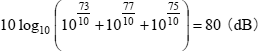Technical Report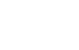What is sound level meter?

11. Octave band analysis (Frequency analysis)

There are two methods for frequency analysis of acoustic vibration: narrow-band analysis (FFT analysis) and octave band analysis.

 Table 11-1: Frequency analysis methods
Analysis band width Typical application
FFT analysis Constant band width Troubleshooting and resolution of physical problems
Octave band analysis Constant band ratio Evaluation of noise and vibration

Octave band analysis is also called CPB (Constant Percentage Bandwidth) analysis. The following paragraphs explain octave band analysis.

11-1 Octave band analysis

To devise noise reduction measures, the noise needs to be analyzed (frequency analysis). One of the noise analysis methods that have been used for many years is octave band analysis. An octave is a series of notes, with the note at the higher end of the series having twice the frequency of the note at the lower end. (Refer to "What is an octave?" below.)

Frequency characteristic felt by the human ear is a geometrical, making octave band analysis a popular means of analysis. The sound pressure level for each band of the noise being measured is determined across the audio frequency range using a bandpass filter meeting 1/1 octave or 1/3 octave standards. For the characteristics of filters and other relevant details, refer to IEC 61260 (JIS C1514:2002).

 What is an octave? An octave is a series of notes, with the note, e.g. ‘do’, at the higher end of the series having twice the frequency of the note, e.g. ‘do’, at the lower end of the series. An octave band is a one-octave range of frequencies straddling across a center frequency, which is called the center octave band frequency. A third of an octave band is called a 1/3 octave band. As shown in the figure on the right, in the 12-tone equal temperament, an octave is divided into 12 parts with constant ratios between the frequencies of any two adjoining tones, with each part called a 1/12 octave band.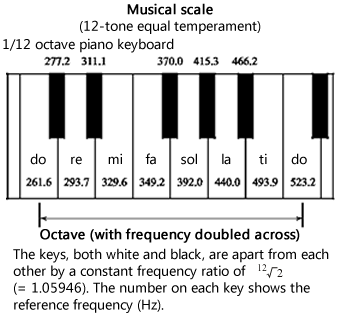11-2 JIS standards on octave filters

There are two JIS standards on octave filters.

1. JIS C 1514:2002 (= IEC 61260:1995) Octave-band and fractional-octave-band filters
2. JIS C 1513:2002 Octave-band and third-octave-band analyzers for sounds and vibrations
1. JIS C1514:2002, which is a translation (IDT) of the corresponding IEC standards, specifies filter characteristics, covering a wide range of filters including not just octave and 1/3 octave filters but 1/N octave filters with required accuracy tolerances of three classes (Class 0, Class 1 and Class 2).

2.

3. JIS C1513:2002 specifies necessary and sufficient specifications for sound and vibration analyzers while making references to the JIS C1514:2002 specifications for the characteristics of octave and 1/3 octave filters. It specifies only Class 1 and Class 2 tolerances, which have steeper characteristics than the conventional Type II and Type III classes. When numbers are smaller, more stringent standards apply.

Table 11-2 shows the center frequencies of octave and 1/3 octave filters. These numbers are the nominal center frequencies specified by ISO 266. The exact center frequencies of JIS-compliant filters are determined using calculations specified in JIS C1514:2002 (IEC 61260:1995).

 Table 11-2: Center frequencies of 1/1 octave and 1/3 octave bandpass filters
Center frequency (Hz) 1/1 octave 1/3 octave Center frequency (Hz) 1/1 octave 1/3 octave Center frequency (Hz) 1/1 octave 1/3 octave
0.8 　 25 　 800
1 31.5 1000
1.25 　 40 　 1250
1.6 　 50 　 1600
2 63 2000
2.5 　 80 　 2500
3.15 　 100 　 3150
4 125 4000
5 　 160 　 5000
6.3 　 200 　 6300
8 250 8000
10 　 315 　 10000
12.5 　 400 　 12500
16 500 16000
20 　 630 　 20000

11-3 Octave filter bandwidth and center frequency

1/1 and 1/3 octaves consist of a number of frequency bandwidths. When:

f1 is the lower cutoff frequency,
f2  is the upper cutoff frequency, and
fm is the center frequency,
then the following equations are true for each octave band.

1/1 octave band filter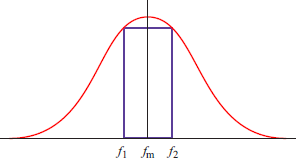(Equation 11-1)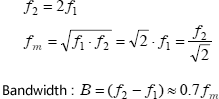Figure 11-1: Center frequency, upper and lower cutoff frequencies and bandwidth of 1/1 octave band

1/3 octave band filter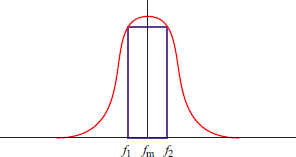(Equation 11-2)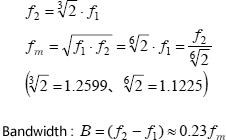Figure 11-2: Center frequency, upper and lower cutoff frequencies and bandwidth of 1/3 octave band

[Supplement]
Center frequency

The following briefly explains how to determine center frequency fm (Source: IEC 61260:1995 (JIS C1514)).

According to IEC 61260 (JIS C1514), exact center frequencies can be determined using the following equations.

1. When N is an odd number (e.g. 1 or 3)

 (Equation 11-3)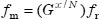• When N is an even number (e.g. 6, 12 or 24)

•  (Equation 11-4)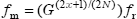....................

where:

 fx : Reference frequency (1000 Hz) x : Integer (positive, negative or 0) N :N of 1/N octave band filter E.g. 1 for 1/1 octave, 3 for 1/3 octave and 6 for 1/6 octave G Number representing octave ratio (nominal frequency ratio of 2:1)

The following two methods are specified for determining G.

 (Equation 11-5) (1) Powers of 10 form: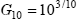(Equation 11-6) (2) Powers of 2 form: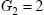By definition, the use of the powers of 2 form should raise no objections. The powers of 10 form was used due to the fact that 10 octaves are almost equal to three decades (210 ≒ 103), which makes G10 in Equation 11-5 around 2 (1.9953). The power of 10 form consists of multiples of 10 and also works well with decimal numbers, making itself a recommended system in standards.

 NOTE: To be exact, the "2" in Equation 11-1 and Equation 11-2 is in fact the G presented above but is used in these equations for convenience and ease of understanding. When the N of a 1/N octave filter is an even number, the reference frequency 1 kHz is not included in the list of center frequencies as is obvious from Equation 11-4.

The following figures show characteristics of 1/1 and 1/3 octave band filters (Source: JIS C1513).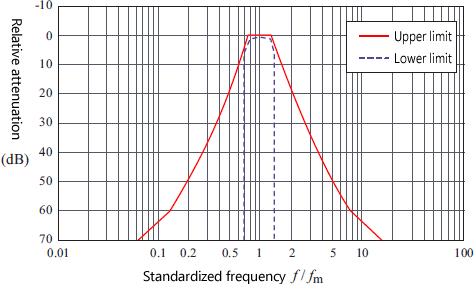Figure 11-3: Relative attenuation limits of Class 1 octave band filter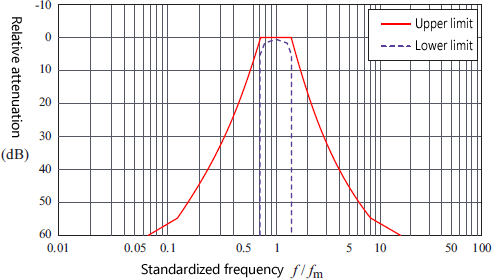Figure 11-4: Relative attenuation limits of Class 2 octave band filter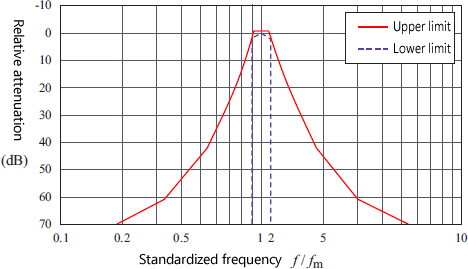Figure 11-5: Relative attenuation limits of Class 1 1/3 octave band filter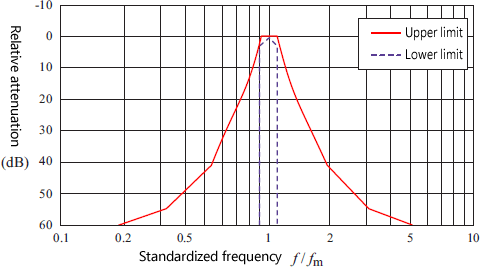Figure 11-6: Relative attenuation limits of Class 2 1/3 octave band filter

[Refernce]
Conversion of 1/3 octave data into 1/1 octave data

When converting known 1/3 octave data in dB into corresponding 1/1 octave data in dB, add up the 1/3 octave data in dB that corresponds to the target 1/1 octave data. For example, when determining the 1/1 octave band data around the center frequency of 1000 Hz and the corresponding 1/3 octave band has the following data in dB,

 800 Hz 73 dB 1000 Hz 77 dB 1250 Hz 75 dB

the 1/1 octave band data around the center frequency of 1000 Hz can be determined using the following equation.

 (Equation 11-7)